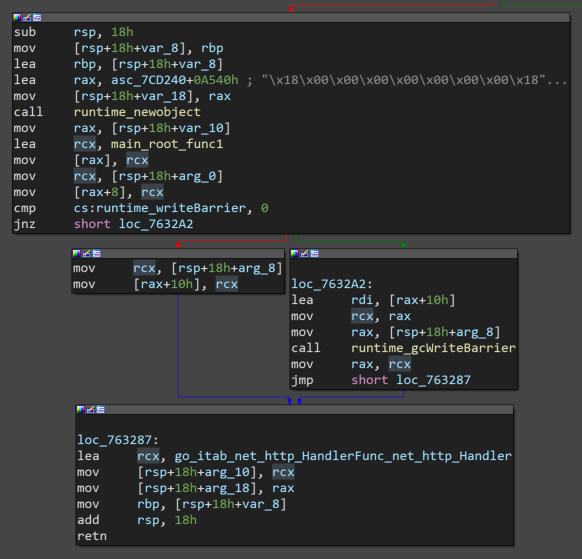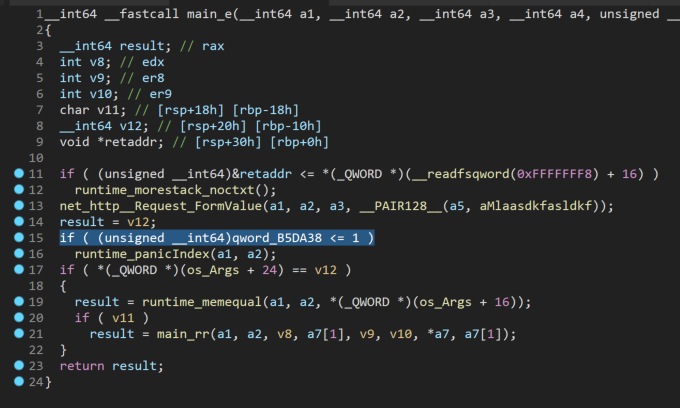# NSEC2020 "Hack the Time" CTF Writeup

## Overview

Note: The challenge binary is available on GitHub.

This is a walk-through of "Hack The Time" a 4-point challenge from the 2020 NSEC CTF. It was a great challenge that required static analysis, dynamic analysis, web skills, Go knowledge, and some creative Bash foo to solve. This was team effort with help from two of my teammates (finding the arg and some bash foo).

Props to @becojo for a great challenge!

## Challenge

`Classes are sooooooo long and I’m bored af. There is a rumour that the student that developed the service that controls the school’s clock added a backdoor to change the time. It is still running to this day… It would be really cool if you can find it :wink: I was able to get a copy of the binary if that helps you: https://dl.nsec/time_server [note binary included in this repo] The school server is running at http://time-server.ctf:8080. `

## Solution

### Web

Browsing to http://time-server.ctf:8080/ displays a simple clock with the current time.

The source of the page is below:

```<!doctype html>
<html lang="en">
<meta charset="UTF-8"/>
<title>Document</title>
<body>
<div id="clock">
<svg viewBox="0 0 40 40">
<circle cx="20" cy="20" r="19" />
<g class="marks">
<line x1="15" y1="0" x2="16" y2="0" />
<line x1="15" y1="0" x2="16" y2="0" />
<line x1="15" y1="0" x2="16" y2="0" />
<line x1="15" y1="0" x2="16" y2="0" />
<line x1="15" y1="0" x2="16" y2="0" />
<line x1="15" y1="0" x2="16" y2="0" />
<line x1="15" y1="0" x2="16" y2="0" />
<line x1="15" y1="0" x2="16" y2="0" />
<line x1="15" y1="0" x2="16" y2="0" />
<line x1="15" y1="0" x2="16" y2="0" />
<line x1="15" y1="0" x2="16" y2="0" />
<line x1="15" y1="0" x2="16" y2="0" />
</g>

<line x1="0" y1="0" x2="9" y2="0" class="hour" />
<line x1="0" y1="0" x2="13" y2="0" class="minute" />
<line x1="0" y1="0" x2="16" y2="0" class="seconds" />
<circle cx="20" cy="20" r="0.7" class="pin" />

<text x="-3" y="0"></text>
</svg>
</div>

<div id="time"></div>

<script src="/script.js"></script>
</body>
</html>```

/script.js:

```async function main() {
var res = await fetch('/time.json');
var {0: j} = await res.json();

document.querySelector('svg text').textContent = j;

var svg = document.querySelector('svg');
var date = new Date(Date.parse(j));

svg.style.setProperty('--start-seconds', date.getSeconds());
svg.style.setProperty('--start-minutes', date.getMinutes());
svg.style.setProperty('--start-hours', date.getHours() % 12);
}

main();

setInterval(main, 5000);```

This script fetches /time.json every 5 seconds. /time.json is an array of the current time, such as:

`["2020-05-16 09:53:24"]`

styles.css just contains CSS to make the clock. I don't have the original CSS due to how I solved the challenge and local testing.

That is the extent of the website; no obvious backdoors exposed. Let's look at the binary.

### Static Analysis

Opening the binary in IDA Pro we see lots of go_ and net_http__ functions.So we have a Go binary. It's a mess, but golang_loader_assist helps resolve function names and strings, and generally makes it a little more pleasant to work with.

You can see in the screenshot above of main.main there are two handlers being registered, /time.json and /, which makes sense given what we saw on the challenge site. There are no other handlers, so the backdoor isn't as easy as /cmd or similar.

Let's look at the /time.json handler (main_timeHandler).It gets the current time (time_Now), formats it (time_Time_Format), coverts it to a string (runtime_convTstring), and finally prints it (fmt_Fprintf). Nothing jumps out as being a backdoor, so let's move on for now. We can come back and reverse engineer this function in more depth if we don't find anything else.

How about main_root the handler for /?Nothing super interesting. Follow the rabbit hole down main_root_func1.Keep going into main_eWell well well. net_http__Request_FormValue with a strange string mlaasdkfasldkfm. This feels like a backdoor. How can we send that string to have it read by FormValue? To the docs!

```// FormValue returns the first value for the named component of the query.
// POST and PUT body parameters take precedence over URL query string values.
// FormValue calls ParseMultipartForm and ParseForm if necessary and ignores
// any errors returned by these functions.
// If key is not present, FormValue returns the empty string.
// To access multiple values of the same key, call ParseForm and // then inspect Request.Form directly. ```

Since we know that / works with GET, we must have to send that string as "name component of the query" aka a GET parameter. The string will be the "key" and whatever it's value is will be returned by FormValue. Something like:

`curl -v http://127.0.0.1:8080/?mlaasdkfasldkfm=test `

Ok so that should get us to the `jbe short loc_76320C` test. What is that checking? Since this is a 64bit ELF, we can see if IDA's pseudocode helps any:If something is <=1 it will panic, but we're not quite sure what that something is. Looking further to line 17, there are two checks against something related to `os_Args` (os_Args + 24 and os_Args +16). If all three of these checks pass, the program will call main_rr. Let's look at main_rr, with a mental note to come back and figure out what these checks are for.As soon as we see os_exec_Command:At this point we're confident we have found the backdoor, but it looks like it has additional checks and it isn't obvious what those checks are for. It's time for dynamic analysis.

### Dynamic Analysis

Before you start any GDB adventures with Go binaries, the Go documentation on GDB integration is required reading.

For this challenge my .gdbinit file contained the following:

```source ~/peda/peda.py
set follow-fork-mode parent # this keeps GDB on the main binary if it forks any children (shells, etc) ```

I am using peda to make GDB a little more friendly.

Let's dig into the binary. First, get it open in GDB: gdb ./time_server

Now we load the function of interest and set a break point at it.

```gdb-peda\$ l main.e
16      in /app/main.go
gdb-peda\$ b main.e
Breakpoint 1 at 0x763160: file /app/main.go, line 21.
gdb-peda\$ r
Starting program: /mnt/hgfs/nsec/time/time_server